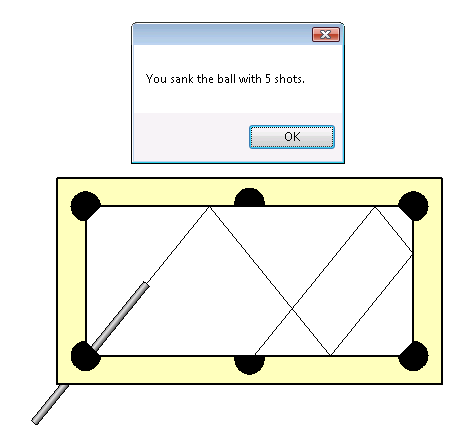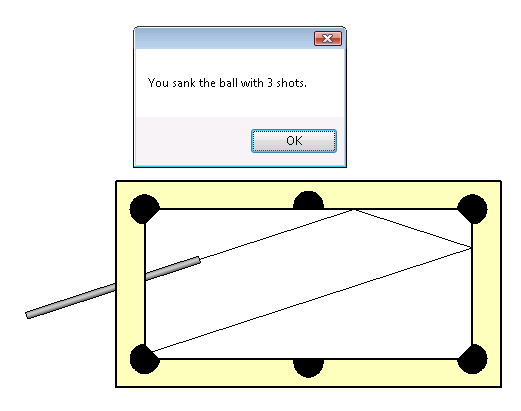### RayTraceBounce Pool Table

In spite of yesterday's promise to stop, here is yet one more last minute Christmas present from Harry Matttison of the Revit API development team. In Harry's own words: "Here is a modification to make the ray shooting SDK example RayTraceBounce a bit more fun ... it works and can amuse a 6 year old, if nothing else."

Harry's sample uses the ray tracing capabilities of the FindReferencesByDirection method to simulate a pool table. The model defines the table, six pockets, and a cue. The cue can be positioned in any way you like. Once positioned, you launch the RaytraceBouncePool command to calculate the walls that the ball will encounter if leaving the cue in a straight line and the reflections off them until it hits a pocket. The number of total reflections required is counted and reported. Here is a shot with four reflections:Here is another one with just two:The command makes use of a couple of handy utility methods:

• Get3DView returns the view named "{3D}" in the current document.
• DeleteLines deletes the lines from the previous run.
• MakeLine creates a model line with the given start point, end point, and line style.

Here is the source code of the entire external command and its utility methods, which Harry placed in the namespace RayTraceBounce:

```public class RaytraceBouncePool : IExternalCommand
{
// have a line style "bounce" created in the
// document before running this

/// <summary>
/// Maximum number of bounces to calculate.
/// </summary>
const int _max_bounces = 100;

/// <summary>
/// Message box caption.
/// </summary>
const string _caption = "RayTraceBounce Pool Table";

ExternalCommandData cdata;
Application app;
Document doc;
Face face = null;
Reference rClosest = null;
View3D view = null;
static double epsilon = 0.00000001;
int LineCount = 0;
int RayCount = 0;

public IExternalCommand.Result Execute(
ExternalCommandData commandData,
ref string message,
ElementSet elements )
{
cdata = commandData;
app = commandData.Application;
doc = app.ActiveDocument;
Get3DView();
DeleteLines();

// find the cue

XYZ direction = null;
XYZ startpt = null;

List<Autodesk.Revit.Element> list
= new List<Autodesk.Revit.Element>();

Filter filter = app.Create.Filter
.NewTypeFilter( typeof( FamilyInstance ) );

int num = app.ActiveDocument.get_Elements(
filter, list );

foreach( Autodesk.Revit.Element e in list )
{
if( e.Name == "cue" )
{
LocationCurve lc = e.Location
as LocationCurve;

direction = lc.Curve.ComputeDerivatives(
0, true ).BasisX;

startpt = lc.Curve.get_EndPoint( 1 );

break;
}
}
if( null == view )
{
MessageBox.Show( "A default 3D view (named {3D}) "
+ " must exist before running this command",
_caption );
}
else
{
for( int ctr = 1; ctr <= _max_bounces; ++ctr )
{
ReferenceArray references
= doc.FindReferencesByDirection(
startpt, direction, view );

rClosest = null;
FindClosestReference( references );

if( rClosest == null )
{
MessageBox.Show( "Ray " + ctr + " aborted. "
+ "No closest face reference found.",
_caption );

return IExternalCommand.Result.Succeeded;
}
else
{
XYZ endpt = new XYZ(
rClosest.GlobalPoint.X,
rClosest.GlobalPoint.Y,
rClosest.GlobalPoint.Z );

if( startpt.AlmostEqual( endpt ) )
{
MessageBox.Show(
"Start and end points are equal. Ray "
+ ctr + " aborted\n"
+ startpt.X + ", "
+ startpt.Y + ", "
+ startpt.Z,
_caption );

break;
}
else
{
MakeLine( startpt, endpt, direction, "bounce" );
RayCount = RayCount + 1;
face = rClosest.GeometryObject as Face;
if( rClosest.Element.Category.Name == "Doors" )
{
MessageBox.Show( "You sank the ball with "
+ ctr + " shots.", _caption );

return IExternalCommand.Result.Succeeded;
}
UV endptUV = rClosest.UVPoint;

// face normal where ray hits

XYZ faceNormal = face.ComputeDerivatives(
endptUV ).BasisZ;

// transformation to get it in terms of
// document coordinates instead of the
// parent symbol

faceNormal = rClosest.Transform.OfVector(
faceNormal );

// http://www.fvastro.org/presentations/ray_tracing.htm

XYZ directionMirrored = direction
- 2 * direction.Dot( faceNormal ) * faceNormal;

// get ready to shoot the next ray

direction = directionMirrored;

startpt = endpt;
}
}
}
}
return IExternalCommand.Result.Succeeded;
}

/// <summary>
/// Find closest reference.
/// </summary>
/// <param name="references">Input array of references</param>
/// <returns></returns>
public Reference FindClosestReference(
ReferenceArray references )
{
double face_prox = Double.PositiveInfinity;
double edge_prox = Double.PositiveInfinity;

foreach( Reference r in references )
{
if( r.Element.Category.Name != "Generic Models" )
{
face = null;
face = r.GeometryObject as Face;
Edge edge = null;
edge = r.GeometryObject as Edge;
if( face != null )
{
// when startpoint is on a surface, should
// FindReferencesByDirection find that surface?

if( Math.Abs( r.ProximityParameter ) < face_prox
&& r.ProximityParameter > epsilon )
{
rClosest = r;
face_prox = Math.Abs( r.ProximityParameter );
}
}
if( edge != null )
{
// when startpoint is on a surface, should
// FindReferencesByDirection find that surface?

if( Math.Abs( r.ProximityParameter ) < edge_prox
&& r.ProximityParameter > epsilon )
{
edge_prox = Math.Abs( r.ProximityParameter );
}
}
}
}

// stop bouncing if there is an edge at least
// as close as the neareast face - there is no
// single angle of reflection for a ray
// striking a line

if( edge_prox <= face_prox )
{
rClosest = null;
}
return rClosest;
}

/// <summary>
/// Create a model line with the given start, end point, and line style.
/// </summary>
/// <param name="sp">Start point</param>
/// <param name="ep">End point</param>
/// <param name="direction">Sketch plane normal vector</param>
/// <param name="style">Line style name</param>
public void MakeLine(
XYZ sp,
XYZ ep,
XYZ direction,
string style )
{
LineCount = LineCount + 1;

Line line = app.Create.NewLineBound( sp, ep );

Plane geometryPlane = app.Create.NewPlane(
direction, sp );

Document doc = app.ActiveDocument;

SketchPlane skplane = doc.Create.NewSketchPlane(
geometryPlane );

ModelCurve mcurve = doc.Create.NewModelCurve(
line, skplane );

ElementArray lsArr = mcurve.LineStyles;

foreach( Autodesk.Revit.Element e in lsArr )
{
if( e.Name == style )
{
mcurve.LineStyle = e;
break;
}
}
}

/// <summary>
/// Return the view named "{3D}" in the current document.
/// </summary>
public void Get3DView()
{
List<Autodesk.Revit.Element> list
= new List<Autodesk.Revit.Element>();

Filter filter = app.Create.Filter.NewTypeFilter(
typeof( View3D ) );

Int64 num = app.ActiveDocument.get_Elements(
filter, list );

foreach( Autodesk.Revit.Element v in list )
{
if( v.Name == "{3D}" )
{
view = v as View3D;
break;
}
}
}

/// <summary>
/// Delete the lines from the previous run.
/// </summary>
public void DeleteLines()
{
List<Autodesk.Revit.Element> list
= new List<Autodesk.Revit.Element>();

Filter filter = app.Create.Filter.NewTypeFilter(
typeof( CurveElement ), true );

Int64 num = app.ActiveDocument.get_Elements(
filter, list );

foreach( Autodesk.Revit.Element e in list )
{
ModelCurve mc = e as ModelCurve;
if( mc != null )
{
if( mc.LineStyle.Name == "bounce"
|| mc.LineStyle.Name == "normal" )
{
app.ActiveDocument.Delete( e );
}
}
}
}
}
```

I integrated this into the standard RayTraceBounce SDK sample code by adding a second external command implementation class named RaytraceBouncePool to the existing project, and the following lines to my RvtSamples.txt file that I use to load all the SDK samples:

```Geometry
RayTraceBounce Pool Table
Simulate a pool table.
LargeImage:
Image:
C:\...\SDK\Samples\RaytraceBounce\RayTraceBounce.dll
RayTraceBounce.RaytraceBouncePool
```

Here is the source file RaytraceBouncePool.cs and the sample project PoolTable.rvt defining the pool table and cue for downloading. I placed the sample project into the RaytraceBounce subdirectory of the SDK Samples folder, and the source file into the CS subdirectory below it.

I hope this helps further understanding of the possibilities of the Revit API and the FindReferencesByDirection method, and provides a bit of fun as well.

Many thanks to Harry for this nice sample!

Once again, and this time for good, a Very Merry Christmas and a Happy New Year to you all!